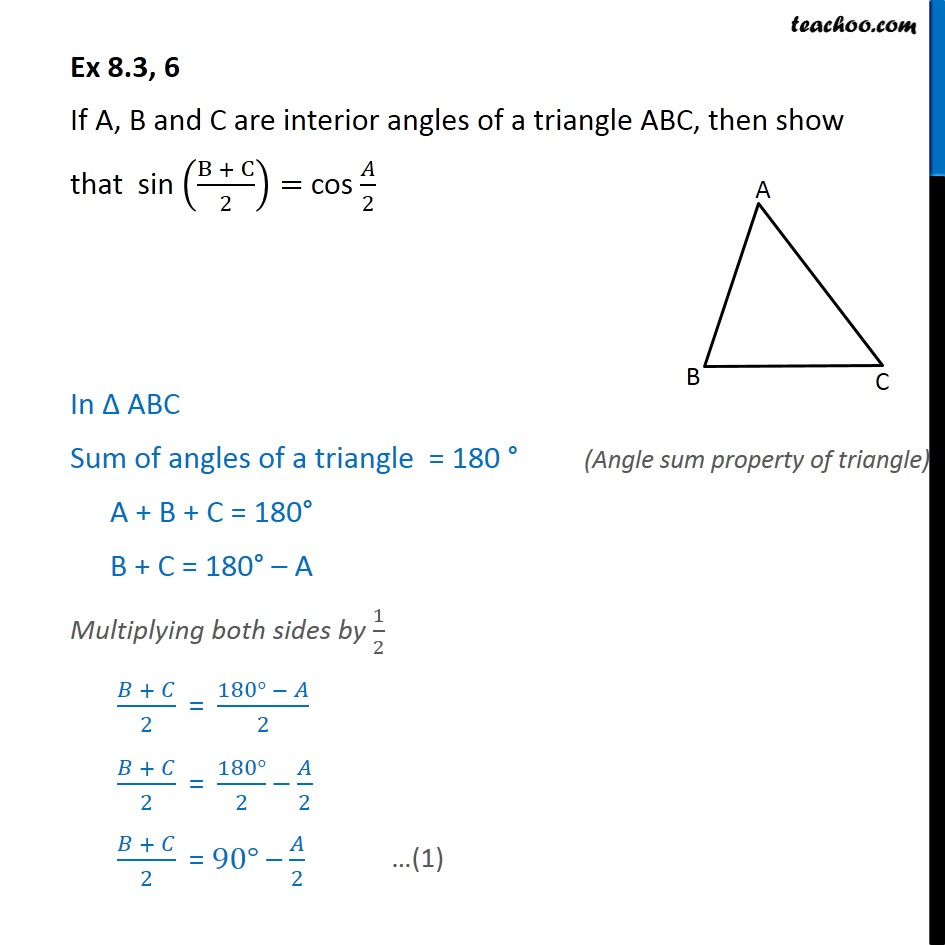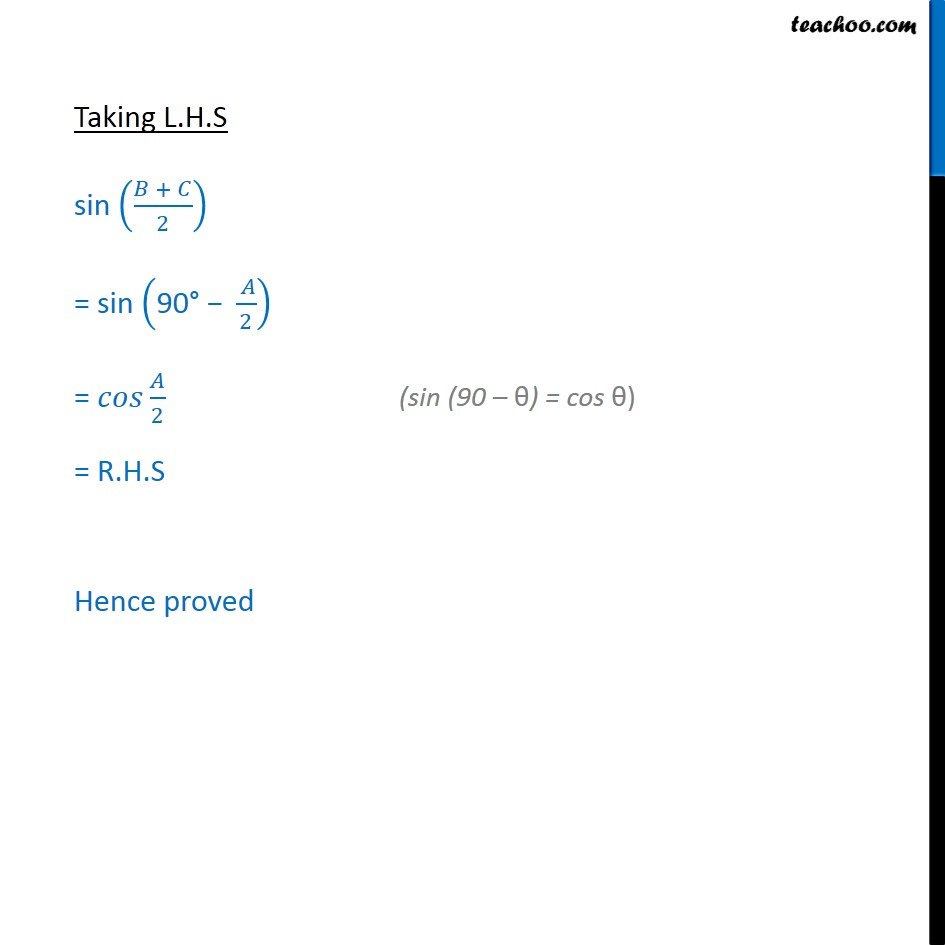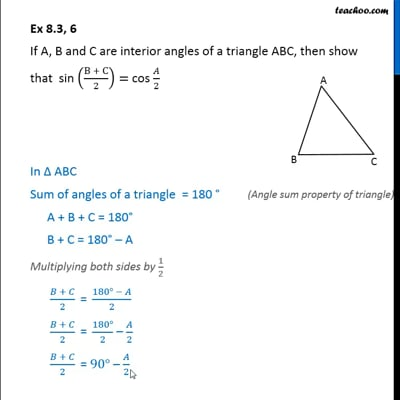Sin 90 - θ, cos 90 - θ formula

Chapter 8 Class 10 Introduction to Trignometry
Serial order wiseThis video is only available for Teachoo black users

Learn in your speed, with individual attention - Teachoo Maths 1-on-1 Class

### Transcript

Question 6 If A, B and C are interior angles of a triangle ABC, then show that sin ((B + C)/2)= cos 𝐴/2 In Δ ABC Sum of angles of a triangle = 180 ° A + B + C = 180° B + C = 180° – A Multiplying both sides by 1/2 (𝐵 + 𝐶)/2 " = " (180° − 𝐴)/2 (𝐵 + 𝐶)/2 " = " (180°)/2 – 𝐴/2 (𝐵 + 𝐶)/2 " = " 90° – 𝐴/2 Taking L.H.S sin ((𝐵 + 𝐶)/2) = sin ("90° − " ( 𝐴)/2) = 𝑐𝑜𝑠 𝐴/2 = R.H.S Hence proved